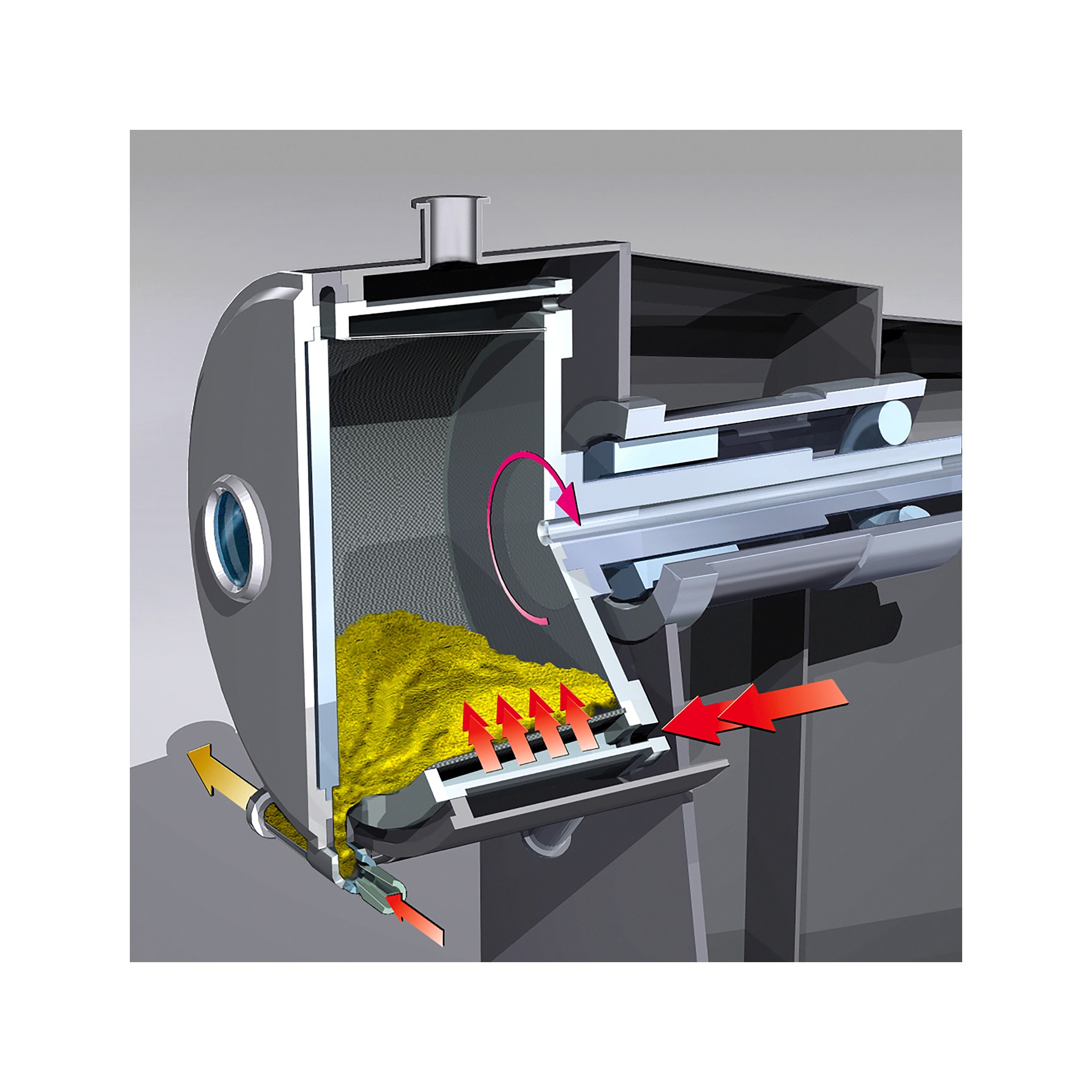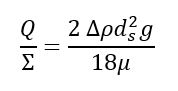# Basic Sizing Equation for Sedimentation Centrifuges

In this post, I want to share basic sizing equation for sedimentation centrifuges. Based on previous post, sedimentation centrifuges used to separate materials based on the density difference between the solid and liquid phases. They are usually used to produce cleared liquid.

Basic sizing equation for sedimentation centrifuges use the term sigma (Σ). The sigma is used to describe a performance of a centrifuge regardless of the physical characteristics of solid-fluid system. The sigma value is equivalent to the cross-sectional area of a gravity settling tank with the same clearing capacity. The sigma value is often expressed in cm2.

The sigma theory is a description of how centrifuge performance is described. It offers a way to compare sedimentation centrifuge performance and to scale up from laboratory and pilot scale experiments.

In the general case, it can be demonstrated that:

Q = 2 ug Σ

and (where Stoke’s law applies):Combining the two equations to get:Note: the factor of 2 is included in equation above as ds is the cut-off size, 50% of particle of this size will be removed in passage through centrifuge.

Where:

Q        = volumetric flow of liquid through the centrifuge (m3/s)

ug       = terminal velocity of the solid particle settling under gravity through the liquid, m/s

Σ        = sigma value of the centrifuge, m2

ρ       = density difference between solid and liquid, kg/m3

ds       = the diameter of the solid particle, the cut-off size, m

μ        = viscosity of the liquid, N.s/m2

g        = gravitational acceleration, 9.8 m/s2

Table below provides selection of the appropriate type of sedimentation centrifuge for certain application based on the ratio of the liquid overflow to sigma value. The table also gives estimation of efficiency rating of each type of operating range. Sigma values are dependent entirely on the geometry and speed of centrifuge.

 Type Approximate Efficiency (%) Normal Operating Range Q, m3/h at Q/Σ m/s Tubular bowl 90 0.4 at 5 × 10-8 to 4 at 3.5 × 10-7 Disc 45 0.1 at 7 × 10-8 to 110 at 4.5 × 10-7 Solid bowl (scroll discharge) 60 0.7 at 1.5 × 10-6 to 15 at 1.5 × 10-5 Solid bowl (basket) 75 0.4 at 5 × 10-6 to 4 at 1.5 × 10-4

Figure below also gives selection guide for sedimentation centrifuges which includes other types of solid-liquid separators.

Let’s see example below.

Example

A precipitate is to be continuously separated from a slurry. The solid concentration is 8% and the slurry feed rate is 6 m3/h. The physical properties at the system operating temperature are:

Liquid density = 1080 kg/m3, viscosity 3.8 cP

Solid density = 2350 kg/m3, “cut-off” particle size 8 μm

Solution

Overflow rate, Q = (100% – 8%) × 6 / 3600 = 1.53 × 10-3 m3/s

ρ = 2350 – 1080 = 1270 kg/m3

Q / Σ = 2 × 1270 × (8 × 10-6)2 × 9.8 / (18 × 3.8 × 10-3) = 2.33 × 10-5

From table above, for a Q 1.53 × 10-3 m3/s at Q / Σ of 2.33 × 10-5 a solid bowl basket type should be used. This type has efficiency of 75% based on the table.

The size of sedimentation centrifuge can be calculated using efficiency value.

Σ = Q / (efficiency × 2 ug) = 1.53 × 10-3 / (2.33 × 10-5 × 75%) = 87.7 m2

The sigma value is the equivalent area of a gravity settler that would perform the same separation as the centrifuge.

I hope you find this post useful.

Reference:

Coulson and Richardson’s Chemical Engineering Volume 6